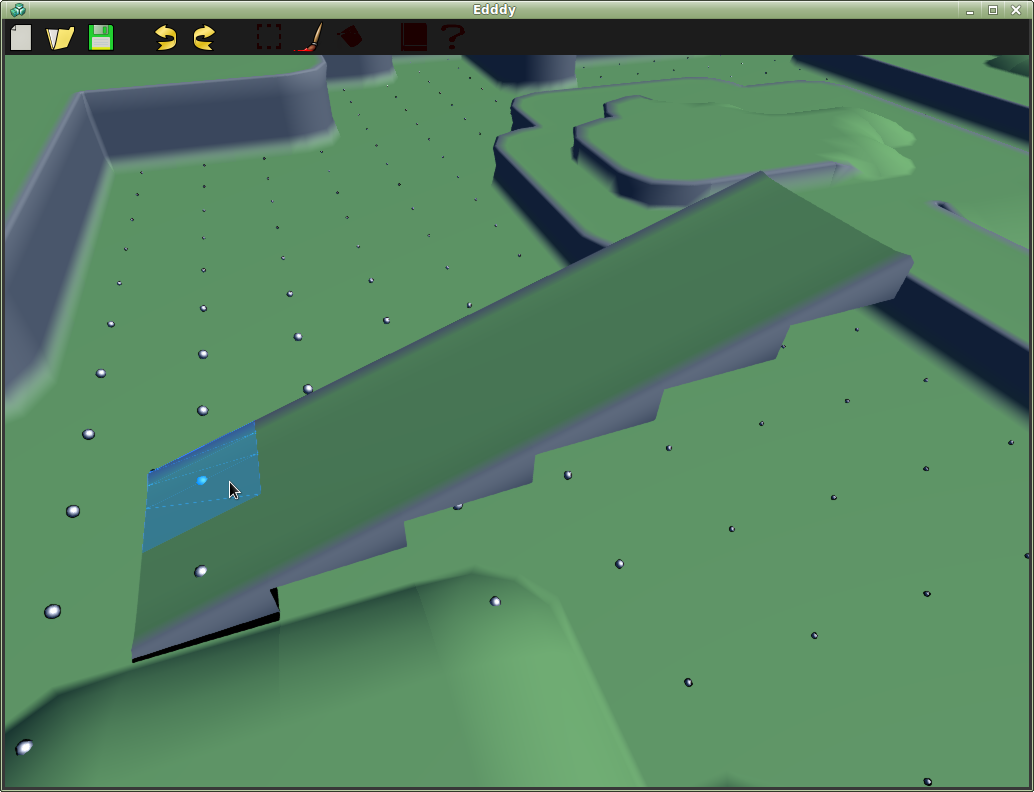{{ message }}

Instantly share code, notes, and snippets.

# yamamushi/bresenham3d

Created Jun 20, 2013
C++ Bresenham 3d Line Drawing Algorithm
 // Bresenham3D // // A slightly modified version of the source found at // http://www.ict.griffith.edu.au/anthony/info/graphics/bresenham.procs // Provided by Anthony Thyssen, though he does not take credit for the original implementation // // It is highly likely that the original Author was Bob Pendelton, as referenced here // // ftp://ftp.isc.org/pub/usenet/comp.sources.unix/volume26/line3d // // line3d was dervied from DigitalLine.c published as "Digital Line Drawing" // by Paul Heckbert from "Graphics Gems", Academic Press, 1990 // // 3D modifications by Bob Pendleton. The original source code was in the public // domain, the author of the 3D version places his modifications in the // public domain as well. // // line3d uses Bresenham's algorithm to generate the 3 dimensional points on a // line from (x1, y1, z1) to (x2, y2, z2) // void Bresenham3D(int x1, int y1, int z1, const int x2, const int y2, const int z2, WorldMap *output, int symbol){ int i, dx, dy, dz, l, m, n, x_inc, y_inc, z_inc, err_1, err_2, dx2, dy2, dz2; int point; point = x1; point = y1; point = z1; dx = x2 - x1; dy = y2 - y1; dz = z2 - z1; x_inc = (dx < 0) ? -1 : 1; l = abs(dx); y_inc = (dy < 0) ? -1 : 1; m = abs(dy); z_inc = (dz < 0) ? -1 : 1; n = abs(dz); dx2 = l << 1; dy2 = m << 1; dz2 = n << 1; if ((l >= m) && (l >= n)) { err_1 = dy2 - l; err_2 = dz2 - l; for (i = 0; i < l; i++) { output->getTileAt(point, point, point)->setSymbol(symbol); if (err_1 > 0) { point += y_inc; err_1 -= dx2; } if (err_2 > 0) { point += z_inc; err_2 -= dx2; } err_1 += dy2; err_2 += dz2; point += x_inc; } } else if ((m >= l) && (m >= n)) { err_1 = dx2 - m; err_2 = dz2 - m; for (i = 0; i < m; i++) { output->getTileAt(point, point, point)->setSymbol(symbol); if (err_1 > 0) { point += x_inc; err_1 -= dy2; } if (err_2 > 0) { point += z_inc; err_2 -= dy2; } err_1 += dx2; err_2 += dz2; point += y_inc; } } else { err_1 = dy2 - n; err_2 = dx2 - n; for (i = 0; i < n; i++) { output->getTileAt(point, point, point)->setSymbol(symbol); if (err_1 > 0) { point += y_inc; err_1 -= dz2; } if (err_2 > 0) { point += x_inc; err_2 -= dz2; } err_1 += dy2; err_2 += dx2; point += z_inc; } } output->getTileAt(point, point, point)->setSymbol(symbol); }

###sriharsha48 commented Dec 11, 2017 • edited

 Hi,What if my coordinates x1.y1,z1 are of type ;double',then I am getting this : error C2296: '<<' : illegal, left operand has type 'double' Can you please explain the way to rectify this error. Also,if I want to use for a size of some 0.3 or 0.5,I want to fix one paramter 'resolution',Which can have any value like 0.3 or 0.5 or 1. I think,your code only works for a grid size of 1,right?

###Modanung commented Dec 18, 2017 • edited

 @sriharsha48 That's what the Bresenham's line algorithm is all about; drawing a line on a grid. From Wikipedia: Bresenham's line algorithm is an algorithm that determines the points of an n-dimensional raster that should be selected in order to form a close approximation to a straight line between two points. It it supposed to only work with integer values... now, let's give it a try. :)

###Modanung commented Dec 18, 2017 • edited

 Seems to be working great! Many thanks @yamamushi!If anyone's interested, the screenshot is from a level editor I'm working on called Edddy.

###yamamushi commented Jan 18, 2018

 @Modanung glad you found it useful! Your level editor looks awesome by the way :)